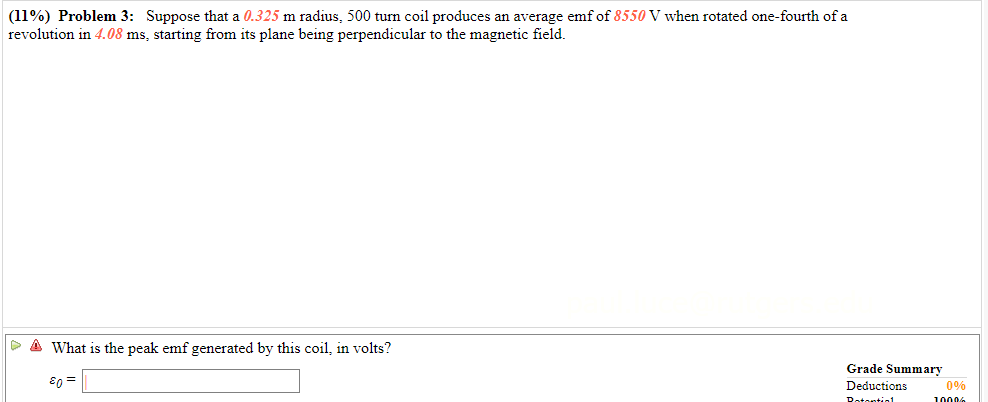# (11%) Problem 3: Suppose that a 0.325 m radius, 500 turn coil produces an average emf of 8550 V when rotated one-fourth of arevolution in 4.08 ms, starting from its plane being perpendicular to the magnetic fieldWhat is the peak emf generated by this coil, in volts?Grade SummaryEn=Deductions0%Rotontio10006

Question
6 viewshelp_outlineImage Transcriptionclose(11%) Problem 3: Suppose that a 0.325 m radius, 500 turn coil produces an average emf of 8550 V when rotated one-fourth of a revolution in 4.08 ms, starting from its plane being perpendicular to the magnetic field What is the peak emf generated by this coil, in volts? Grade Summary En= Deductions 0% Rotontio 10006 fullscreen
check_circle

Step 1

Given:

Radius of the coil = 0.325 m

Number of turns = 500

Average emf, εavg  = 8550 V

Time = 4.08 ms

Step 2

Εavg can be given as:

Step 3

Distance covered in 1/4th o...

### Want to see the full answer?

See Solution

#### Want to see this answer and more?

Solutions are written by subject experts who are available 24/7. Questions are typically answered within 1 hour.*

See Solution
*Response times may vary by subject and question.
Tagged in

### Physics